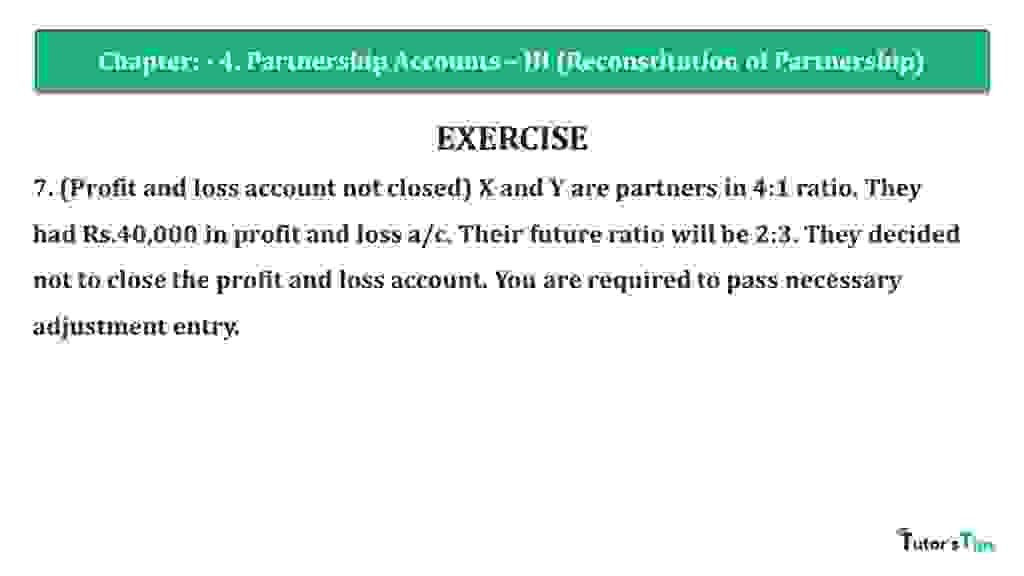# Question 07 Chapter 4 of +2 Part-1 – USHA Publication 12 Class Part – 1Question 07 Chapter 4 of +2-Part-1

7. (Profit and loss account not closed) X and Y are partners in 4:1 ratio. They had Rs.40,000 in profit and loss a/c. Their future ratio will be 2:3. They decided not to close the profit and loss account. You are required to pass necessary adjustment entry.

### The solution of Question 07 Chapter 4 of +2 Part-1: –

 In the Books of _______________ Date Particulars L.F. Debit Credit Y’s Capital A/c Dr. 16,000 To X’s Capital A/c 16,000 (Being profit and loss adjusted)

Working Notes: –

 Old Ratio of X and Y = 4: 1 New Ratio of X and Y, = 2 : 3

Calculate the Sacrificing or Gaining Ratio of Partners
Sacrificing or Gaining Ratio = Old Ratio – New Ratio

 X’s Share Sacrificing/Gaining = 4 – 2 5 5
 = 4 – 2 5
 = 2 (Sacrificing) 5
 Y’s Share Sacrificing/Gaining = 1 – 3 5 5
 = 1 – 3 5
 = -2 (Gaining) 5

 Amount of profit & loss Credited to X’s Capital = 40,000 X 2 5 = 16,000

 Amount of profit & loss debited to Y’s Capital = 40,000 X 2 5 = 16,000